# 79. Parametrised Surface 1. Consider the parametrised surface defined by: x 2u, y u2+ v (a) Find a vector normal to the...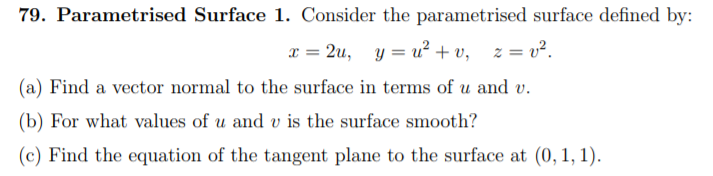79. Parametrised Surface 1. Consider the parametrised surface defined by: x 2u, y u2+ v (a) Find a vector normal to the surface in terms of u and v. (b) For what values of u and v is the surface smooth? (c) Find the equation of the tangent plane to the surface at (0,1, 1)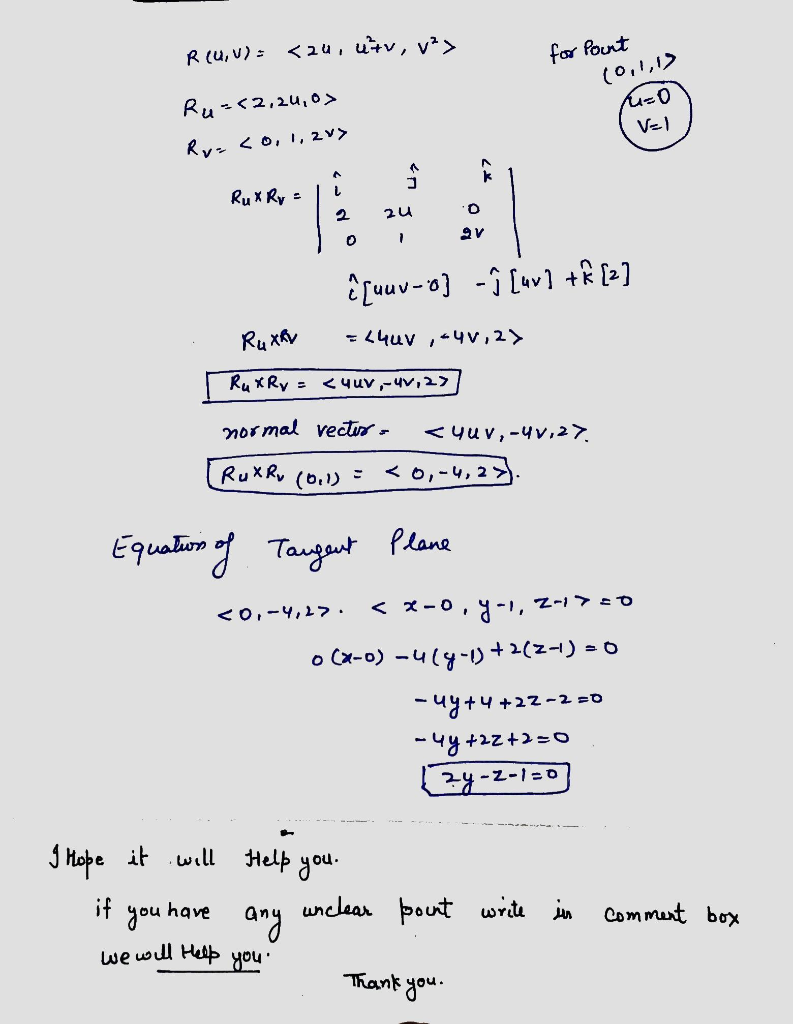##### Add Answer of: 79. Parametrised Surface 1. Consider the parametrised surface defined by: x 2u, y u2+ v (a) Find a vector normal to the...
Similar Homework Help Questions
• ### Unit normal vector to level curveConsider the function (a) Find the unit normal vector to the level curve passing through the point (0,1)(b) Find the equation of the tangent plane to the surface z=f(x,y) when x=0 and y=1Please show all steps

• ### unit normal vector and tangent plane

Consider the function f(x,y) = 3(x^4)y - (2(x^2)(y^2)) + (y^4)a. find the unit normal vector to the level curve passing through the point (0,1)b. find the equation of the tangent plane to the surface z = f(x,y) when x=0 and y=1

• ### Consider the surface given as a graph of the function g(x, y) = x∗y 2 ∗cos(y). The gradient of g ...

Consider the surface given as a graph of the function g(x, y) = x∗y 2 ∗cos(y). The gradient of g represents the direction in which g increases the fastest. Notice that this is the direction in the xy plane corresponding to the steepest slope up the surface, with magnitude equal to the slope in that direction. 1. At the point (2, π), find the gradient, and explain what it means. 2. Use it to construct a vector in the tangent...

• ### 12. A surface is given parametrically by the equations -u2-u y lue, = (3u-20)". Find an...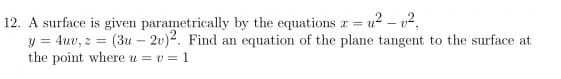12. A surface is given parametrically by the equations -u2-u y lue, = (3u-20)". Find an equation of the plane tangent to the surface at the point where u u = 1

• ### Given f(x, y): 10-2x2-y, find a) The equation of the tangent plane to the surface at the point (2...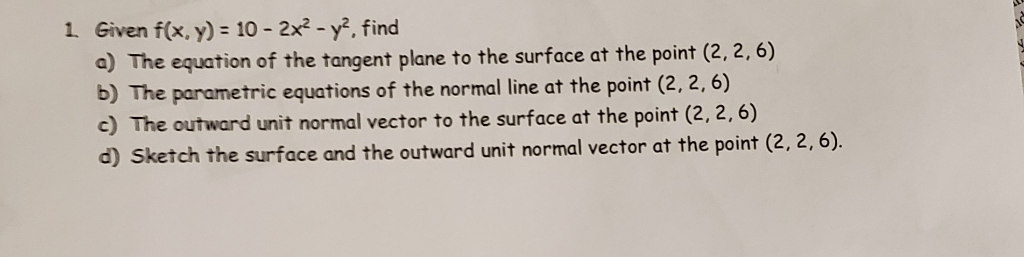Given f(x, y): 10-2x2-y, find a) The equation of the tangent plane to the surface at the point (2.2,6) b) The parametric equations of the normal line at the point (2, 2, 6) c) The outward unit normal vector to the surface at the point (2, 2,6) d) Sketch the surface and the outward unit normal vector at the point (2, 2,6). 1. Given f(x, y): 10-2x2-y, find a) The equation of the tangent plane to the surface at the...

• ### TOTAL MARKS: 25 QUESTION 4 (a) Find a normal vector and an equation for the tangent plane to the ...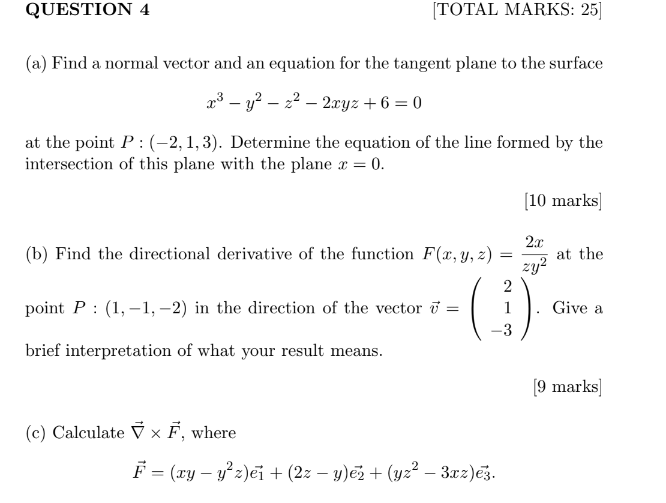TOTAL MARKS: 25 QUESTION 4 (a) Find a normal vector and an equation for the tangent plane to the surface at the point P: (-2,1,3). Determine the equation of the line formed by the intersection of this plane with the plane z = 0. 10 marks (b) Find the directional derivative of the function F(r, y, z)at the point P: (1,-1,-2) in the direction of the vector Give a brief interpretation of what your result means. 2y -3 [9 marks]...

• ### Question 2. Consider a surface S in the 0 plane with three smooth boundary curves C1, C2, and C3 as shown in the diagram. Each curve is parametrised so that it is traversed in the direction shown by...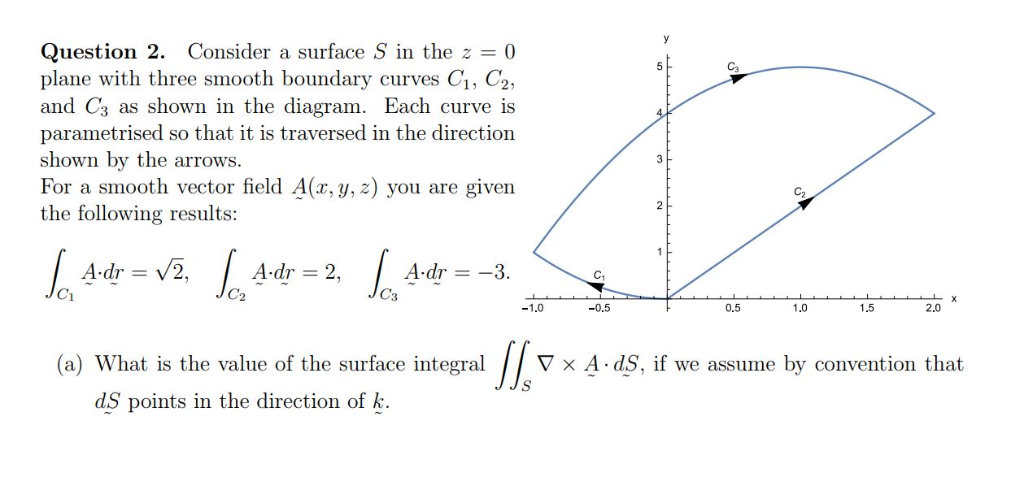Question 2. Consider a surface S in the 0 plane with three smooth boundary curves C1, C2, and C3 as shown in the diagram. Each curve is parametrised so that it is traversed in the direction shown by the arrows For a smooth vector field A(x, y, z) you are given the following results: Ca Adr =-3 C2 0.5 2.0 1.5 -0.5 (a) What is the value of the surface integral ▽ × A. ds. if we assume by convention...

• ### 5. Suppose σ is a parametric surface with vector equation r(14. u) x (u, u)i + y(u, u)j + z(u, v)...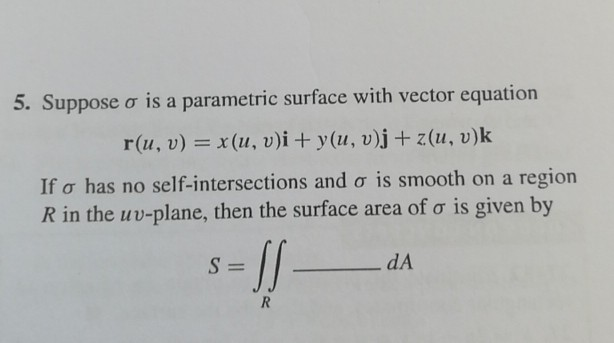5. Suppose σ is a parametric surface with vector equation r(14. u) x (u, u)i + y(u, u)j + z(u, v)k If σ has no self-intersections and σ 1s smooth on a region R in the uu-plane, then the surface area of ơ is given by 5. Suppose σ is a parametric surface with vector equation r(14. u) x (u, u)i + y(u, u)j + z(u, v)k If σ has no self-intersections and σ 1s smooth on a region R...

• ### all questions are related and need help answering! rough the surface 4. o pm) What is the value of the flux of the vector field F(x,y)j+z ioriented with upward- pointing normal vector? (A) 0 (B)...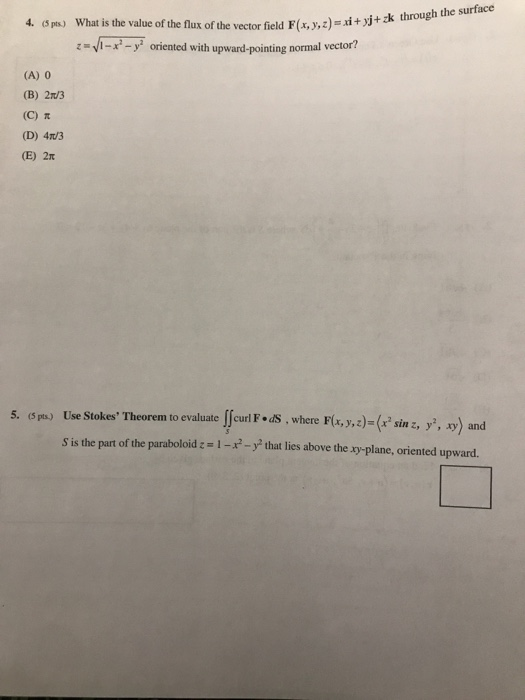all questions are related and need help answering! rough the surface 4. o pm) What is the value of the flux of the vector field F(x,y)j+z ioriented with upward- pointing normal vector? (A) 0 (B) 2n/3 (C) π (D) 4T/3 (E) 2π Use Stokes, Theorem to evaluateⅡcurl F.dS, where F(x, y, z)-(x2 sin Theorem to evaluate Jceun F'.asS , where Fl.e)(', ») and 5. (5pts.) F,y, sin z, y', xy) and s is the part of the paraboloid : -...

• ### Find a normal vector and an equation for the tangent plane to the surface: x3 - y2 - z2 - 2xyz + 6 =0 at the point P : (−2, 1, 3). Determine the equation of the line formed by the intersection of this...

Find a normal vector and an equation for the tangent plane to the surface: x3 - y2 - z2 - 2xyz + 6 =0 at the point P : (−2, 1, 3). Determine the equation of the line formed by the intersection of this plane with the plane x = 0. [10 marks] (b) Find the directional derivative of the function F(x, y, z) = 2x /zy2 , at  the point P : (1, −1, −2) in the direction of...

Need Online Homework Help?